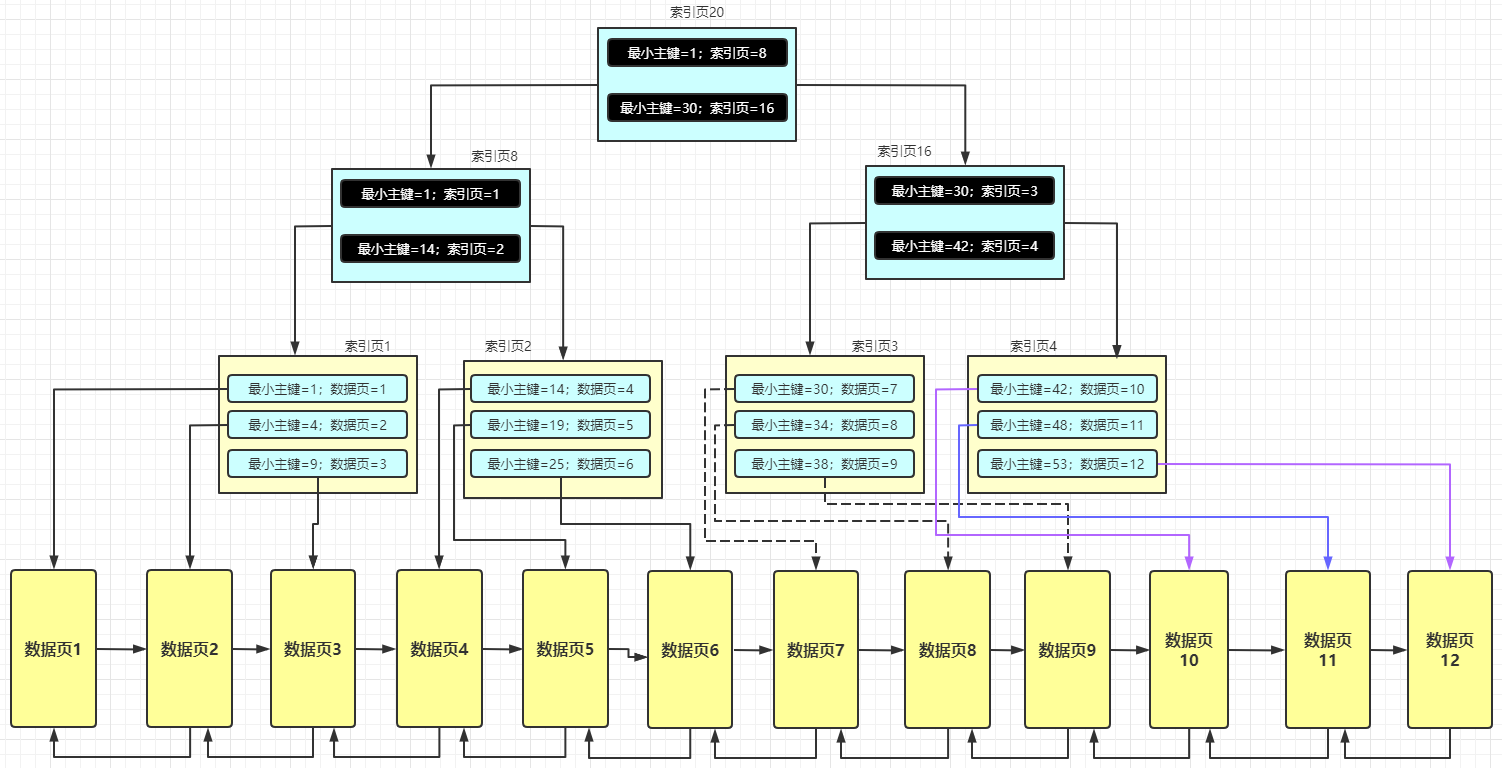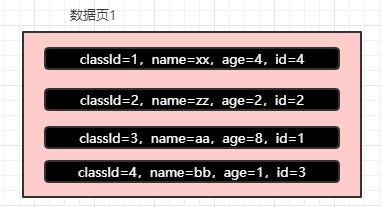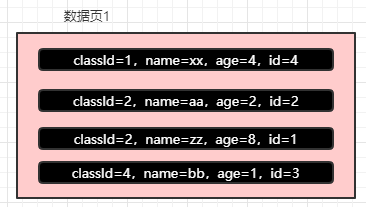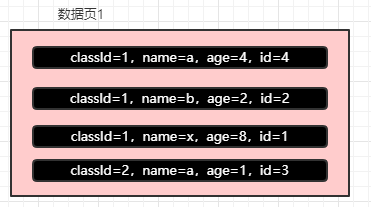# 再有人问你MySQL是如何查询数据的，请把这篇文章甩给他！

GitHub 19k Star 的Java工程师成神之路，不来了解一下吗！

MySQL 对于主键索引的维护是最简单的，就是根据主键去维护一个 B+ 树，因为主键的特点一般是递增的，也就是说是有序的，所以 MySQL 在维护的时候只需要将记录依次往数据页中追加即可，数据页满了就继续添加到下一个数据页。且每一条记录是完整的，即所有的列的值都维护。## 1、等值匹配原则

``````SELECT * FROM student WHERE name='wx' AND age=1
``````

## 2、最左前缀匹配原则

``````classId=1,name=wx,age=1,id=1;
classId=1,name=xq,age=2,id=2;
classId=1,name=wx,age=1,id=3;
classId=2,name=zs,age=3,id=4;
``````

``````SELECT * FROM student WHERE  age=1
``````

## 3、范围查找规则

``````SELECT * FROM student WHERE classId > 1 AND classId < 4
``````

``````SELECT * FROM student WHERE classId > 1 AND classId < 4 and name > a AND name < x
``````## 4、等值匹配+范围查找

``````SELECT * FROM student WHERE classId = 1 AND name > a AND name < x
``````## 5、Order By + limit 优化

``````SELECT name,age,address FROM student ORDER BY  name,age,address LIMIT 10
``````

## 6、分组查询优化

``````SELECT count(*) FROM student GROUP BY NAME
``````

## 7、结束语

MySQL 是如何帮我们维护非主键索引的

age 字段值还一样，就根据主键字段排序。

(全文完)### 评论 1

1. #1

第5条的，说索引覆盖的，这句话是不是错了：
那么此时就需要再去进行回表到聚簇索引中查询了。

gogo7891年前 (2021-03-19)回复

## HollisChuang's Blog

• 微信咨询
• 去评论
• 回顶
###### 回顶部